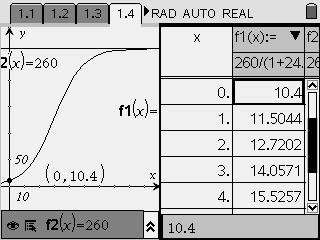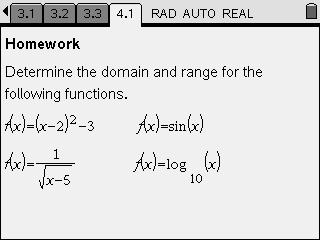# Activities

••• ##### Subject Area

• Math: Precalculus: Exponential and Logarithmic Functions
• Math: Precalculus: Logistic Functions

• ##### Author9-12

15 Minutes

• ##### Device
• TI-Nspire™
• TI-Nspire™ CAS
• ##### Software

TI-Nspire™
TI-Nspire™ CAS

1.7

## Domain & Range#### Activity Overview

A variety of mathematical functions and real world applications of functions are explored to help students learn the concepts of domain and range, as well as how the context of a problem impacts domain and range.

#### Key Steps

•Students find the domain and range of various real-world problems. The first problem involves exploring an equation that models the height of a sunflower plant over time.

•There are extra problems at the end of the activity for students to complete either in class or for homework. These problems include sine, logarithmic, quadratic, square root functions.